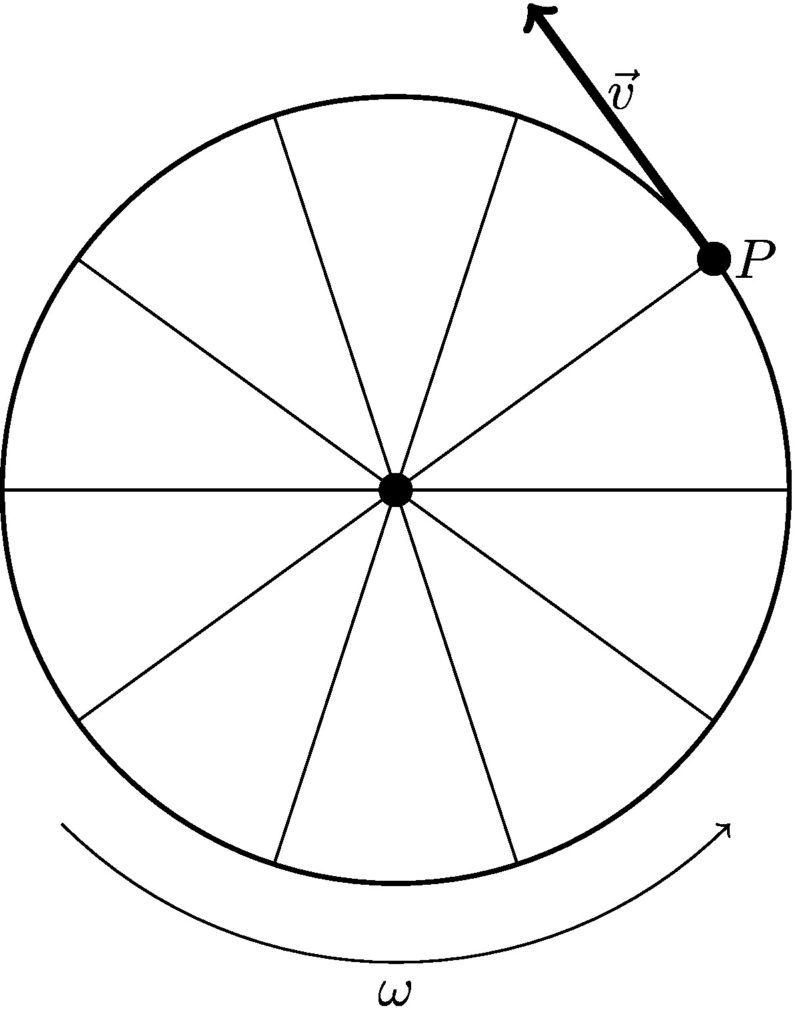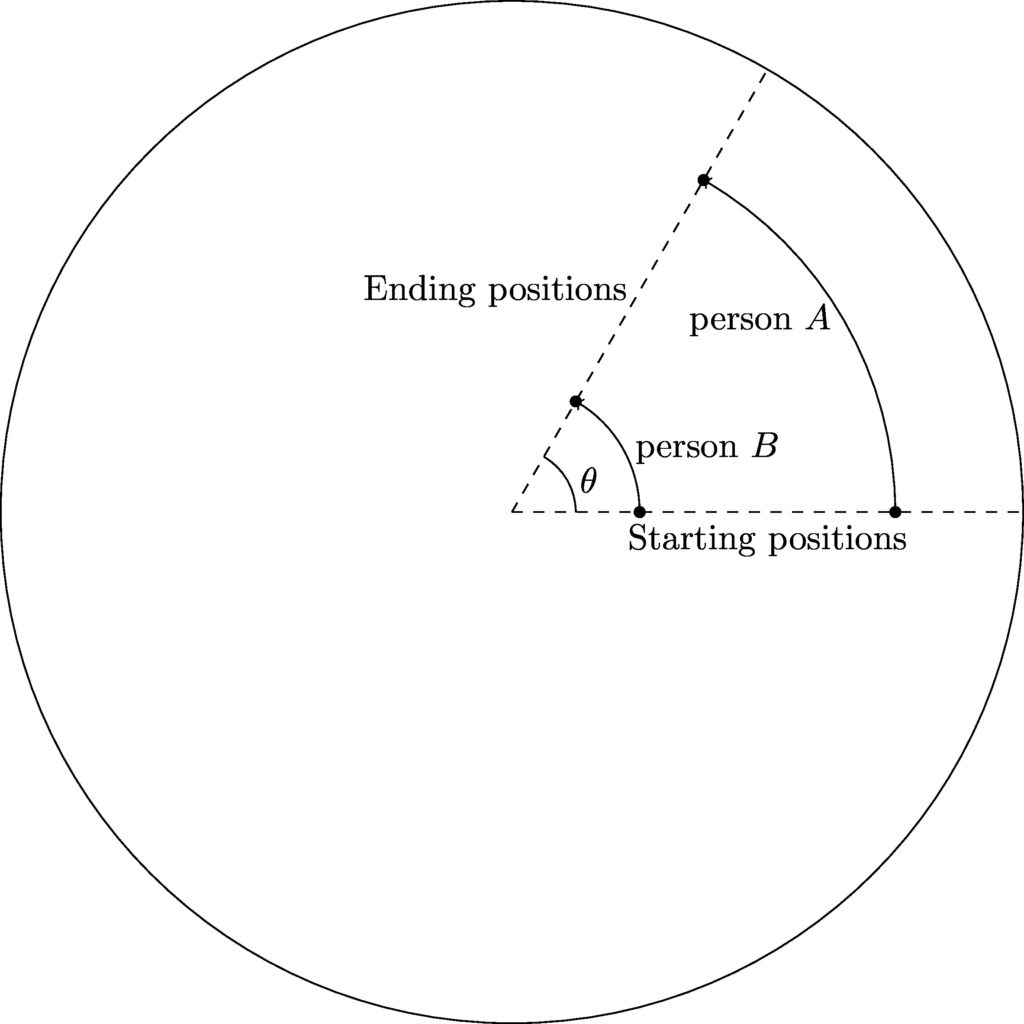# Chapter 5: Rotational motion

## 5.2 Connecting translation & rotation

### 5.2.1 Tangential speed

Consider an object traveling at some speed $$v$$ in a circular path, such as a ball being swung around on a string. At any given instant, an object moving along a circular path has some velocity pointing in a direction tangential to the path (see the figure below). This is called the tangential velocity, $$\vec{v}_t$$. Since the object is traveling in a circle, the direction is constantly changing. However, the magnitude of the tangential velocity—called the tangential speed—is given by:

$v_t = r\omega \tag{5.5}$

This has units of meters per second. The tangential speed is what an instrument such as a baseball pitching coach’s radar gun would tell you, if used to measure the motion of an object moving in a circular path.An object traveling in a circular path has a velocity that is directed tangent to the circle.

#### Example 5.2

The radius of the Earth at the equator is 6378 km. Given that Earth makes one rotation each day, find the tangential speed of a person at the equator.

Use the relationship between tangential speed, angular speed, and radius:

\begin{align*} v_t &= r\omega \\ &= \left(6378\ \textrm{km}\right)\left(\frac{2\pi\ \textrm{rad}}{24\ \textrm{hr}}\right) \\ &= 1670\ \textrm{km/hr} \end{align*}

That’s about a thousand miles per hour!

#### Example 5.3

You and your friend are riding on a merry-go-round. If your friend is twice as far from the center of the merry-go-round as you are, how many times faster are they traveling?

Since you are both on the same rotating object, your angular speed is the same. In the relationship $$v_t = r\omega$$, we see that if you double $$r$$ and keep $$\omega$$ the same, you double $$v_t$$. (When two variables are related like this they are said to be directly proportional.) So, your friend is traveling twice as fast as you are.

Notice that a point on farther from the axis of rotation is actually traveling faster than a point closer in. Think about it this way: in the time it takes to make one complete rotation, your friend (who is closer to the outer edge of the merry-go-round) travels a much larger distance than you. The circumference of your friend’s circular path is larger than that of yours. Since your friend traveled a larger distance in the same amount of time, they are traveling at a higher speed. The figure below shows how the length of the paths around a circle differ depending on distance from the center.A person on the outside of a merry go round has a higher tangential speed than someone closer to the center, because they travel a larger distance in the same amount of time.

### 5.2.2 Rolling motion

We have been talking about rotation around a fixed axis. But what if the axis of rotation was moving forward, like a bicycle wheel as you ride in a straight line? The motion of the wheel is a combination of rotational and translational motion.

The figure above shows a wheel with a radius $$R$$. If the wheel rolls without slipping, as it makes one complete revolution it moves forward by a distance equal to the circumference. It does this in some time $$T$$. The speed of the axle is then
$v = \frac{2\pi R}{T}$
Since it takes time $$T$$ to make one complete rotation of $$2\pi$$ radians, $$\frac{2\pi}{T}$$ is the angular speed of the wheel. This gives us
$v = R\omega$